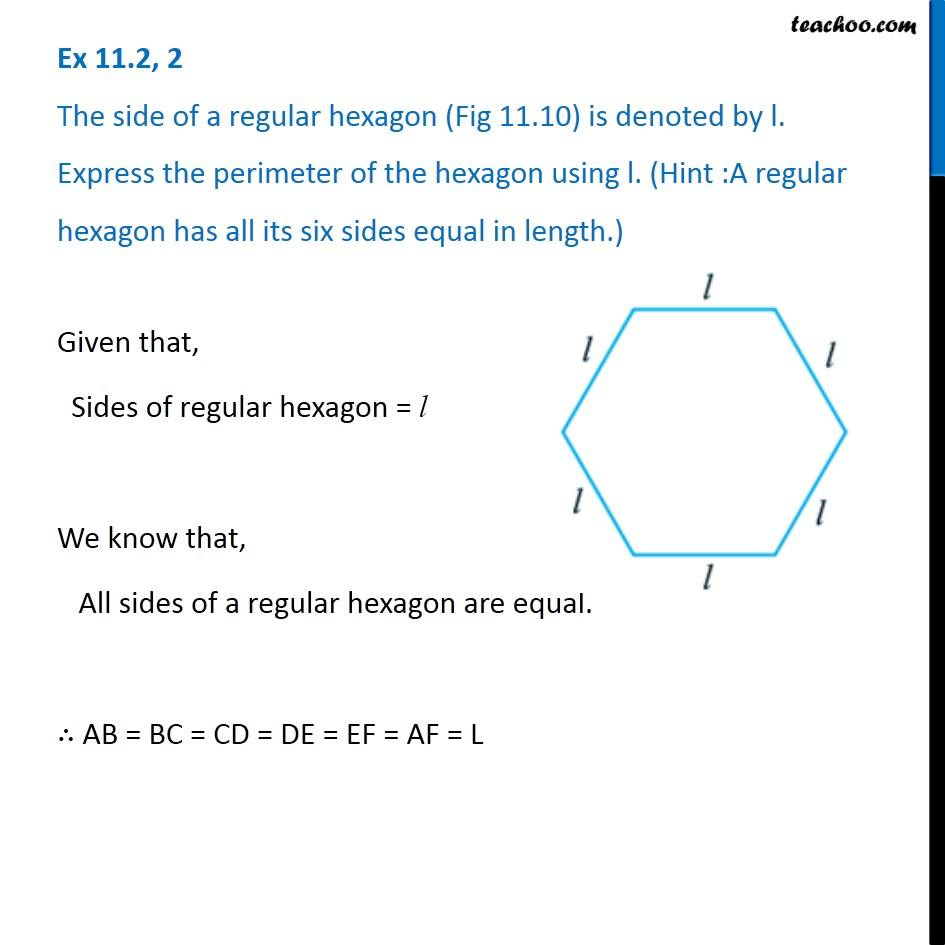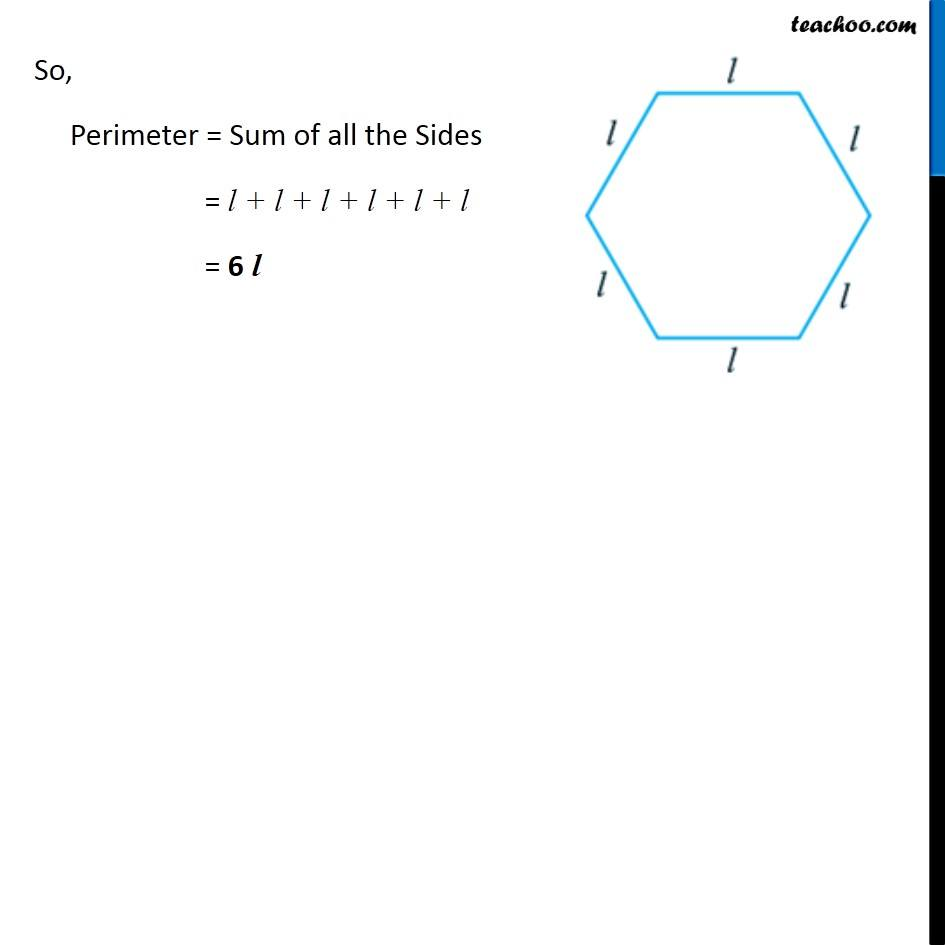Perimeter as variable

Chapter 11 Class 6 Algebra
Concept wiseLearn in your speed, with individual attention - Teachoo Maths 1-on-1 Class

### Transcript

Question 2 The side of a regular hexagon (Fig 11.10) is denoted by l. Express the perimeter of the hexagon using l. (Hint :A regular hexagon has all its six sides equal in length.) Given that, Sides of regular hexagon = l We know that, All sides of a regular hexagon are equal. ∴ AB = BC = CD = DE = EF = AF = L So, Perimeter = Sum of all the Sides = l + l + l + l + l + l = 6 l